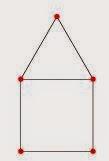## Tuesday, 19 August 2014

### Chromatic Properties of House and House X Graphs

As with Bull Graphs, House Graphs are simple graphs (not the Graph Theoretic definition) which have been neglected. They may not be interesting or yield any important findings to professional research mathematicians, but I haven't been able to find a paper or website which lists or shows any of the House Graphs properly at all. My intention is simply to state and prove of these properties.

Please note I'm not a professional mathematician and I'm not even studying for a Mathematics degree (Computer Science), and therefore may make mistakes or misunderstandings.

There are two main variants of House Graphs, and in this article I will listing the Vertex Colouring and Edge Colouring properties of such graphs. The two graphs are the House Graph and the House-X Graph.House GraphHouse-X Graph
Both of the graphs are simple graphs, and the same number of vertices. In terms of the structure of the graph, the only difference between the graphs are the number of edges. The number edges for the House Graph is 6 and the number of edges for the House-X Graph is 8. The number of edges will be fundamental to the chromatic properties of these graphs.

Chromatic Number:

The Chromatic Number is defined to be the minimum vertex colouring of a graph G. $$\chi(G)$$ is the common notation for the chromatic number of a given graph called G. The minimum vertex colouring is the smallest number of k-colours needed to colour the vertices, so that no two adjacent vertices contain the same colour; no two vertices connected by a common edge have the same vertex colour.
The chromatic number of a House X Graph is 4, this is very obvious, because the chromatic number of a complete graph with n vertices is n. The House-X Graph contains a complete graph of 4 vertices, and the addition of a 'isolated' single vertex creates a chromatic number of 4.

The chromatic number of the House Graph is 3, for a one main reason, the 3-Complete Graph is a subgraph of the House Graph which leads to the fact at least three colours to required. This isn't really a rigorous proof as such, but by attempting to colour the graph with only 2 colours, the idea will become more apparent.

Chromatic Index:

The Chromatic Index of a graph is defined to be the minimum edge colouring of a graph G. The edge colouring of a graph, is when two or more edges incident to a common vertex do not share the same edge colour. Since the House-X Graph and the House Graph are both simple graphs, then Vizing's Theorem can be applied here to show the chromatic index of both graphs.
Vizing's Theorem states that the minimum number of colours needed to edge colour a graph is the maximum degree or the maximum degree with the addition of 1. The maximum degree of a graph is the largest degree of a vertex within the graph. The degree is the number of edges incident to that vertex.

The House Graph has a chromatic index of 3, and the House-X Graph has a chromatic index of 4. The chromatic index for the House Graph is mostly due to the 3-Complete Graph being present as a subgraph, and the fact that Complete Graphs on odd n vertices will have a chromatic index of n.

Reference(s):